Economic Growth Economic growth is growth of the

• Slides: 23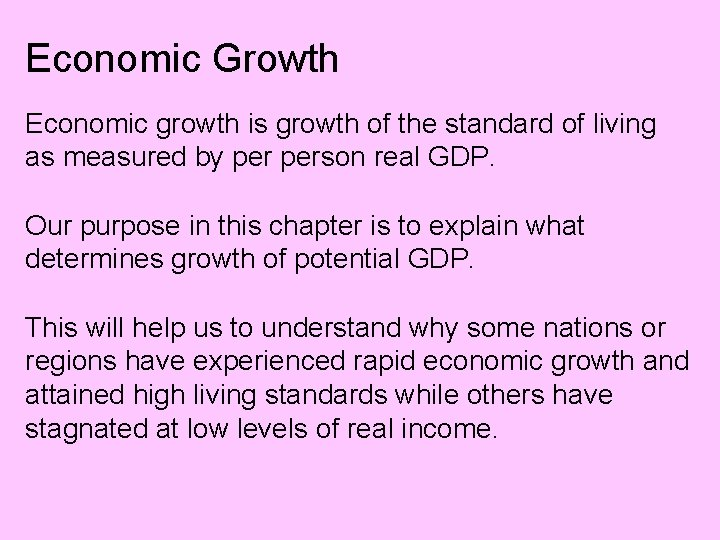Economic Growth Economic growth is growth of the standard of living as measured by person real GDP. Our purpose in this chapter is to explain what determines growth of potential GDP. This will help us to understand why some nations or regions have experienced rapid economic growth and attained high living standards while others have stagnated at low levels of real income.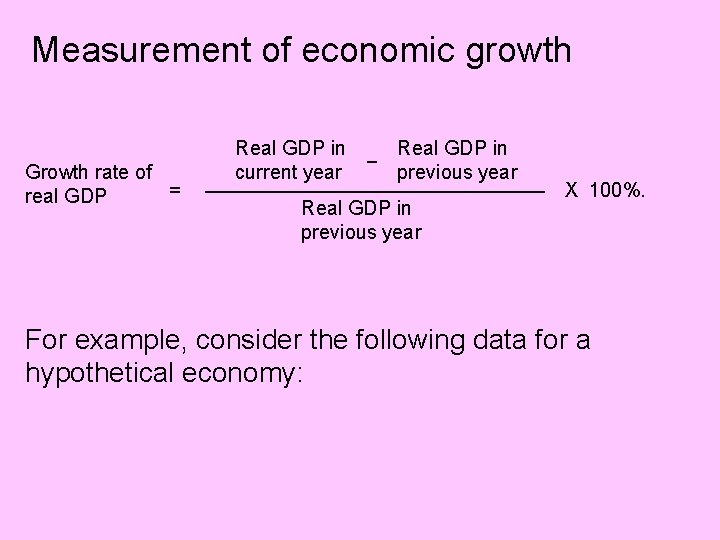Measurement of economic growth Growth rate of = real GDP Real GDP in current year _ Real GDP in previous year _________________ Real GDP in previous year X 100%. For example, consider the following data for a hypothetical economy: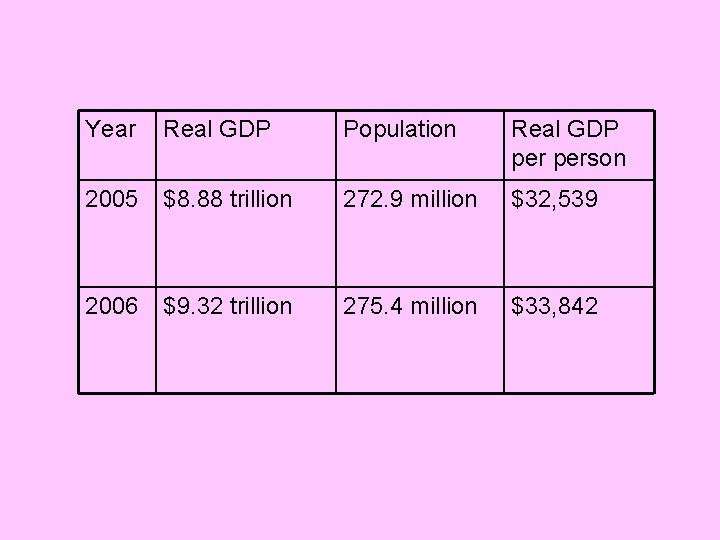Year Real GDP Population Real GDP person 2005 \$8. 88 trillion 272. 9 million \$32, 539 2006 \$9. 32 trillion 275. 4 million \$33, 842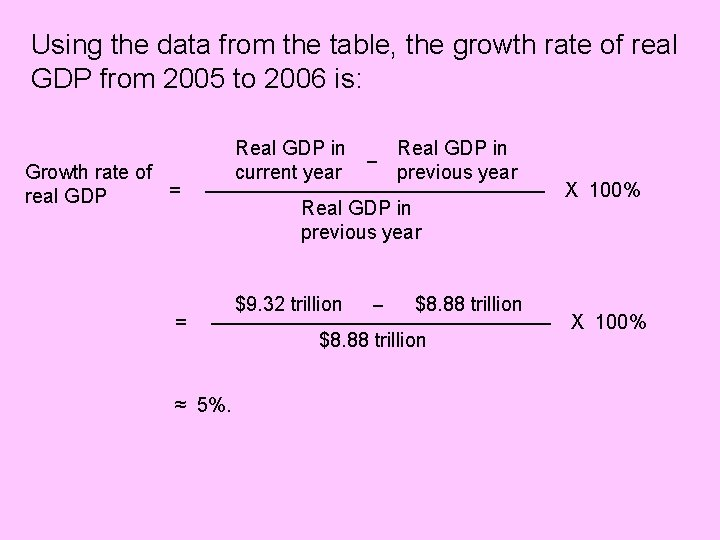Using the data from the table, the growth rate of real GDP from 2005 to 2006 is: Growth rate of = real GDP = Real GDP in current year _ Real GDP in previous year _________________ Real GDP in previous year _ \$8. 88 trillion _________________ ≈ 5%. \$9. 32 trillion \$8. 88 trillion X 100%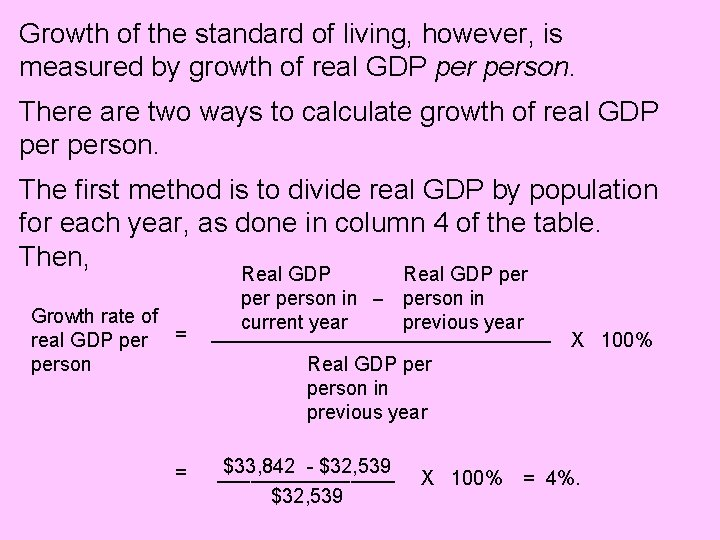Growth of the standard of living, however, is measured by growth of real GDP person. There are two ways to calculate growth of real GDP person. The first method is to divide real GDP by population for each year, as done in column 4 of the table. Then, Growth rate of real GDP per = person = Real GDP per person in _ person in current year previous year _________________ X 100% Real GDP person in previous year \$33, 842 - \$32, 539 ________ \$32, 539 X 100% = 4%.The second method of calculating the growth rate of real GDP person is as follows: Growth rate of real GDP person = Growth rate of real GDP _ Growth rate of population. Using the data from our table, the growth rate of the population from 2005 to 2006 is given by _ 275. 4 million 272. 9 million X 100% _____________ 272. 9 million ≈ 1%. Recall that the growth rate of real GDP was approximately 5%. Therefore, Growth rate of real GDP person = 5% - 1% = 4%.The rule of 70 Growth works like compound interest: If you deposit \$100 in a bank account at a 5% annual interest rate, at the end of the year you will have \$100 + \$5. If you leave the money in the bank for another year, you will earn interest on the \$100 and interest on the \$5. Because you are earning “interest on interest, ” over a period of years the initial deposit of \$100 will compound to a larger amount. How many years will it take for the \$100 to double to \$200? We use the “rule of 70” to estimate the answer.The rule of 70 states that the number of years it takes for the level of any variable to double is approximately 70 divided by the annual percentage growth rate of the variable. Thus, if the annual interest rate on our \$100 deposit is 5%, this means the \$100 grows at an annual rate of 5%. Therefore the \$100 will double to \$200 in 70 ___ = 14 years. 5 The same approach can be applied to economic growth: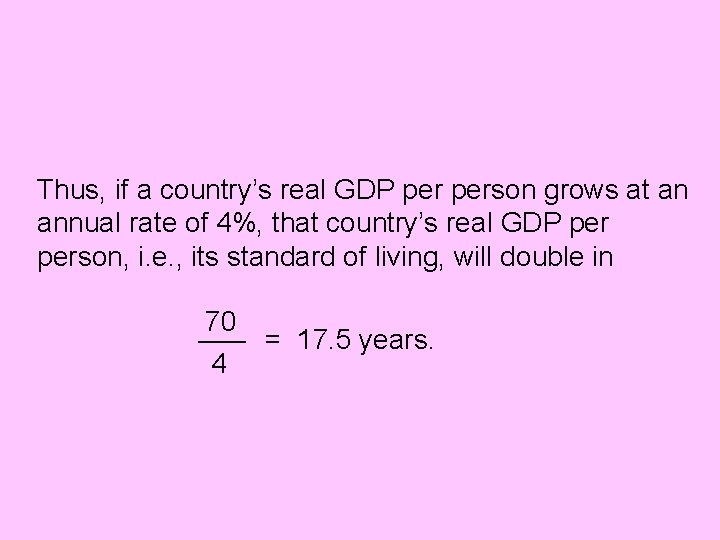Thus, if a country’s real GDP person grows at an annual rate of 4%, that country’s real GDP person, i. e. , its standard of living, will double in 70 ___ = 17. 5 years. 4Sources of economic growth Real GDP increases if we employ more labor. Consider the aggregate production function: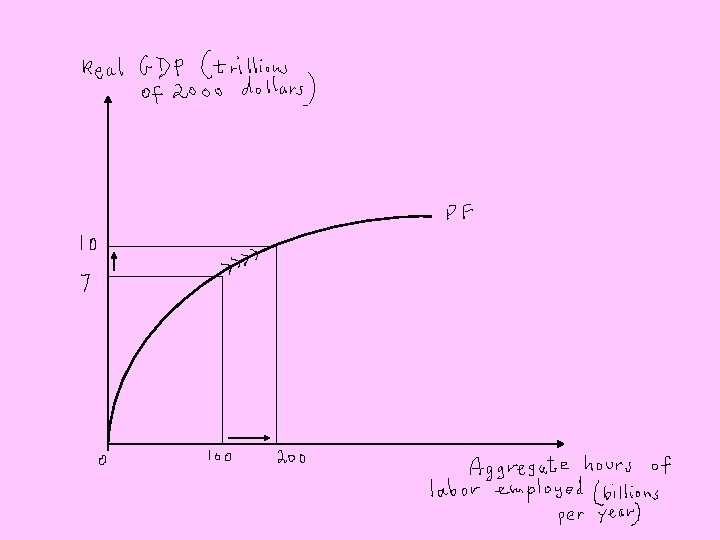Real GDP increases from \$7 trillion to \$10 trillion when we increase aggregate hours of labor employed from 100 billion to 200 billion per year. Aggregate hours of labor employed can increase due to an increase in average hours the labor force participation rate population But average hours worked per worker has actually decreased over time. And although the labor force participation rate has increased, it clearly has an upper limit.This leaves population growth as the only source of growth in aggregate labor hours that is sustainable over time. But although population growth brings growth of real GDP, it does not necessarily bring growth of real GDP person, i. e. , growth of the standard of living, unless labor becomes more productive. Thus, our standard of living will improve only if we produce more goods and services (more real GDP) with each hour of labor. If a given amount of labor produces more real GDP, this means that the aggregate production function has shifted up: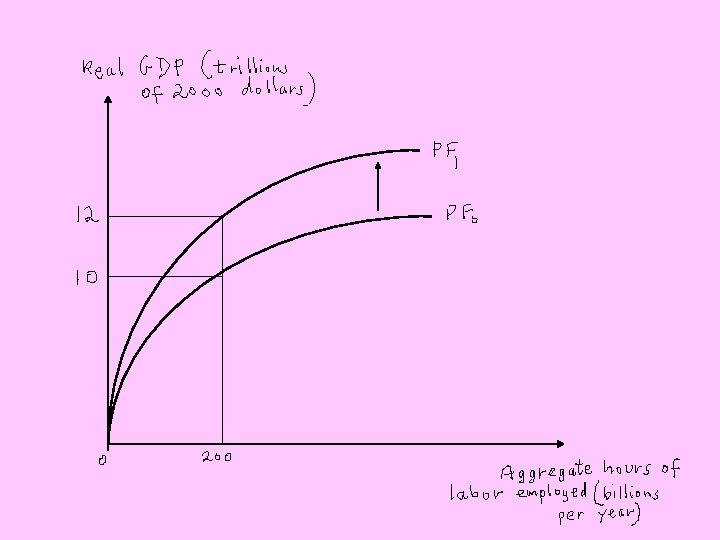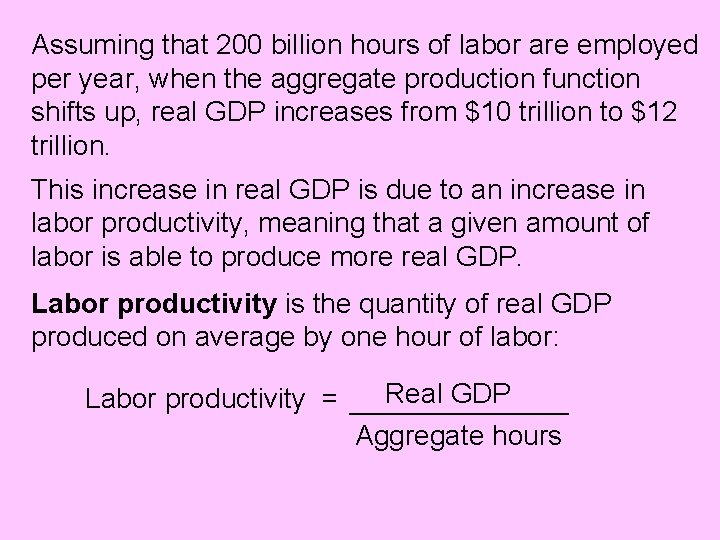Assuming that 200 billion hours of labor are employed per year, when the aggregate production function shifts up, real GDP increases from \$10 trillion to \$12 trillion. This increase in real GDP is due to an increase in labor productivity, meaning that a given amount of labor is able to produce more real GDP. Labor productivity is the quantity of real GDP produced on average by one hour of labor: Labor productivity = Real GDP ___________ Aggregate hours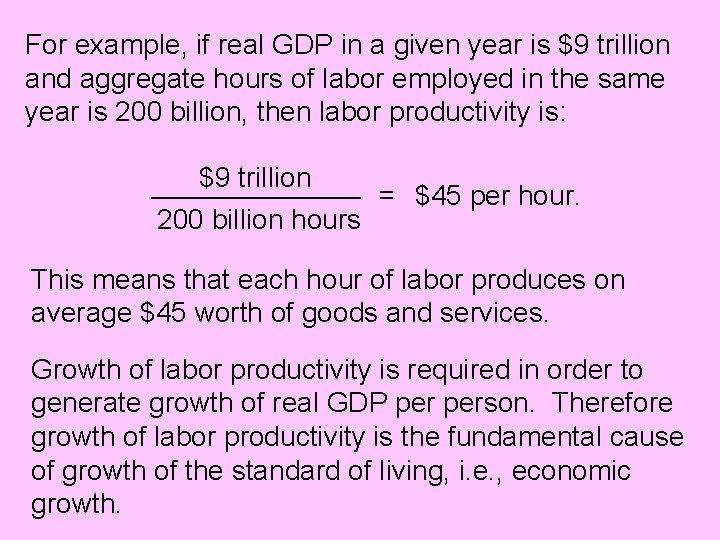For example, if real GDP in a given year is \$9 trillion and aggregate hours of labor employed in the same year is 200 billion, then labor productivity is: \$9 trillion ___________ 200 billion hours = \$45 per hour. This means that each hour of labor produces on average \$45 worth of goods and services. Growth of labor productivity is required in order to generate growth of real GDP person. Therefore growth of labor productivity is the fundamental cause of growth of the standard of living, i. e. , economic growth.Growth of labor productivity is caused by: increases in the physical capital stock increases in the stock of human capital technological innovation Increases in physical capital and human capital require investment expenditures on plant and equipment, in the case of physical capital, and education and training, in the case of human capital. These investment expenditures, in turn, require saving in order to provide the funds for investment. Efficient financial markets are necessary to channel saving into productive investment expenditures.Growth of human capital not only directly increases labor productivity, but is also the source of discovery of new technologies which, historically, has been the most important contributor to growth of labor productivity. To take advantage of new technologies, physical and human capital must increase. For example, firms must invest in new computers to benefit from the invention of a new, faster microprocessor; and individual workers or their employers must invest in new skills to be able to use a new technology.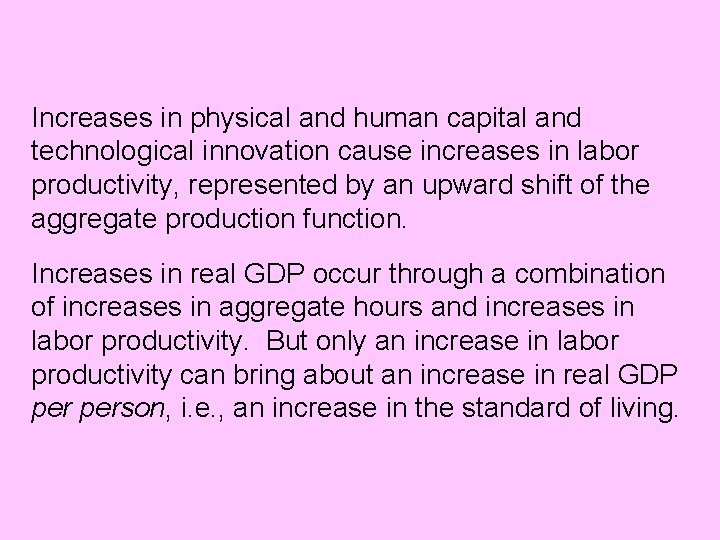Increases in physical and human capital and technological innovation cause increases in labor productivity, represented by an upward shift of the aggregate production function. Increases in real GDP occur through a combination of increases in aggregate hours and increases in labor productivity. But only an increase in labor productivity can bring about an increase in real GDP person, i. e. , an increase in the standard of living.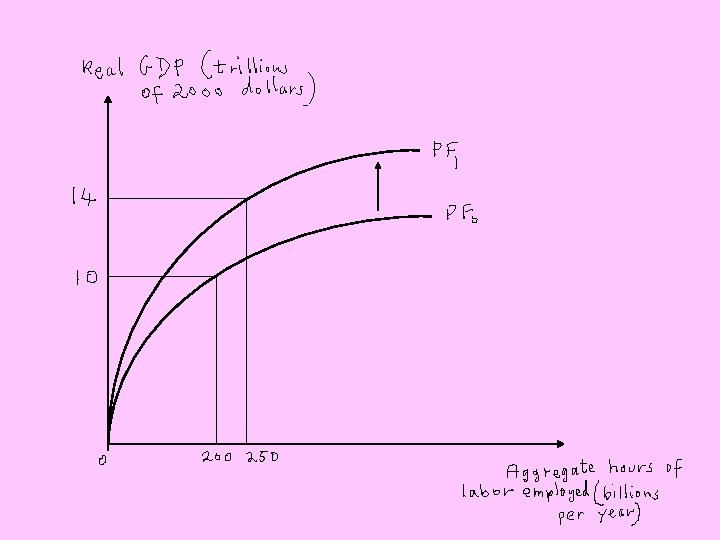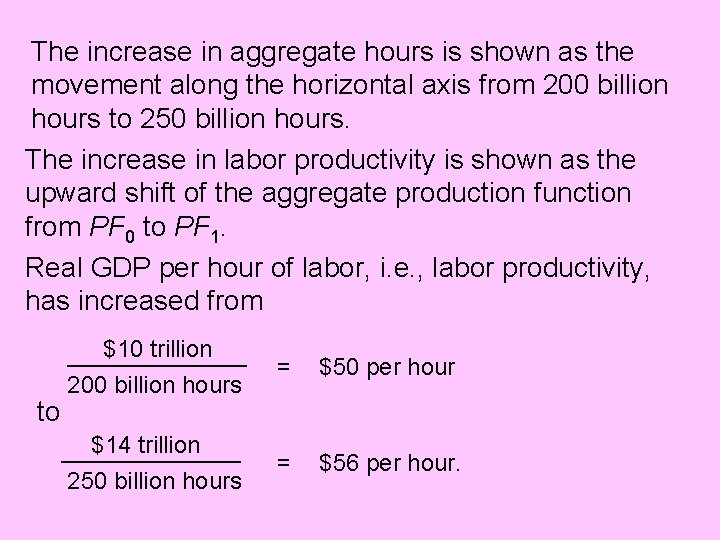The increase in aggregate hours is shown as the movement along the horizontal axis from 200 billion hours to 250 billion hours. The increase in labor productivity is shown as the upward shift of the aggregate production function from PF 0 to PF 1. Real GDP per hour of labor, i. e. , labor productivity, has increased from \$10 trillion _________ to 200 billion hours \$14 trillion _________ 250 billion hours = \$50 per hour = \$56 per hour.Preconditions for economic growth What conditions are necessary for economic growth? We have seen that growth of real GDP person requires saving and investment to increase the stock of physical capital, investment in human capital to enhance the skills of the labor force, and technological innovation. A necessary condition for all of these is economic freedom, which is freedom to exchange in markets and enforcement of private property rights. Property rights and markets create incentives for individuals to specialize, trade, save, invest, expand their human capital, and discover new technologies.No country with a high level of economic freedom is poor. But many countries that lack economic freedom stagnate at low levels of person real GDP. Thus, as Adam Smith pointed out in his 1776 book, The Wealth of Nations, the main cause of the wealth of nations, i. e. , the main determinant of economic growth, is having the right institutions.